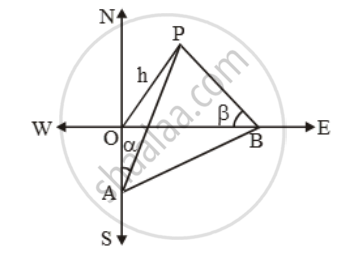Share

# The angle of elevation of the top of a tower from a point A due south of the tower is α and from B due east of the tower is β. If AB = d, show that the height of the tower is - CBSE Class 10 - Mathematics

#### Question

The angle of elevation of the top of a tower from a point A due south of the tower is α and from B due east of the tower is β. If AB = d, show that the height of the tower is

\frac{d}{\sqrt{\cot ^{2}\alpha +\cot^{2}\beta

#### Solution

Let OP be the tower and let A and B be two points due south and east respectively of the tower such that ∠OAP = αand ∠OBP = β.

Let OP = h. In ∆OAP, we have\tan \alpha =\frac{h}{OA}

⇒ OA = h cot α ….(i)

In ∆OBP, we have

\tan \beta =\frac{h}{OB}

⇒ OB = h cot β. ….(ii)

Since OAB is a right angled triangle. Therefore,

AB^2 = OA^2 + OB^2

⇒ d^2 = h^2 cot^2 α + h^2 cot^2 β

\Rightarrow h=\frac{d}{\sqrt{\cot ^{2}\alpha +\cot ^{2}\beta )

[Using (i) and (ii)]

Is there an error in this question or solution?

#### Video TutorialsVIEW ALL 

Solution The angle of elevation of the top of a tower from a point A due south of the tower is α and from B due east of the tower is β. If AB = d, show that the height of the tower is Concept: Heights and Distances.
S